Probability Theory Probability Experiment Outcome Event Defined PROBABILITY

• Slides: 13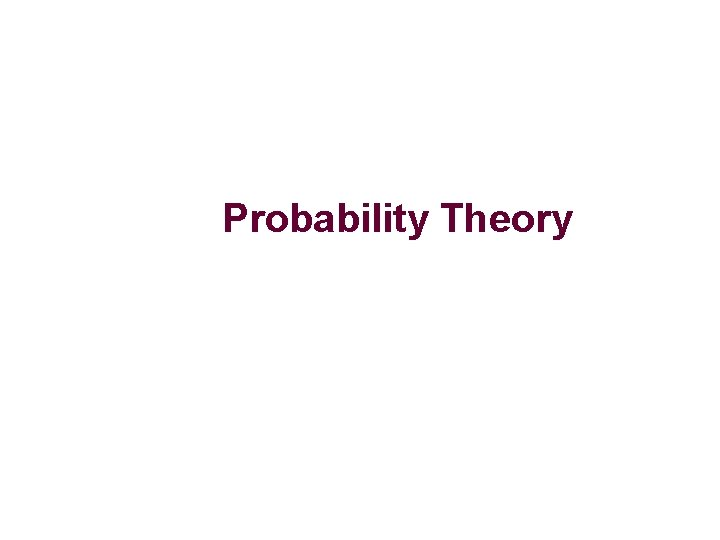Probability Theory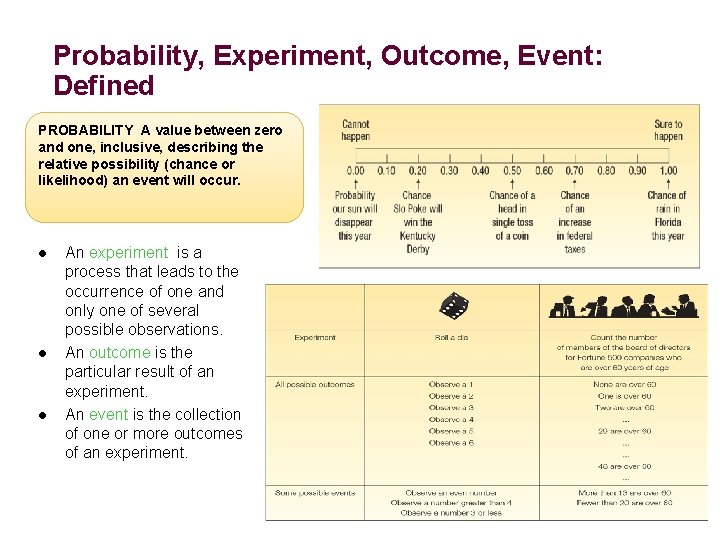Probability, Experiment, Outcome, Event: Defined PROBABILITY A value between zero and one, inclusive, describing the relative possibility (chance or likelihood) an event will occur. l l l An experiment is a process that leads to the occurrence of one and only one of several possible observations. An outcome is the particular result of an experiment. An event is the collection of one or more outcomes of an experiment.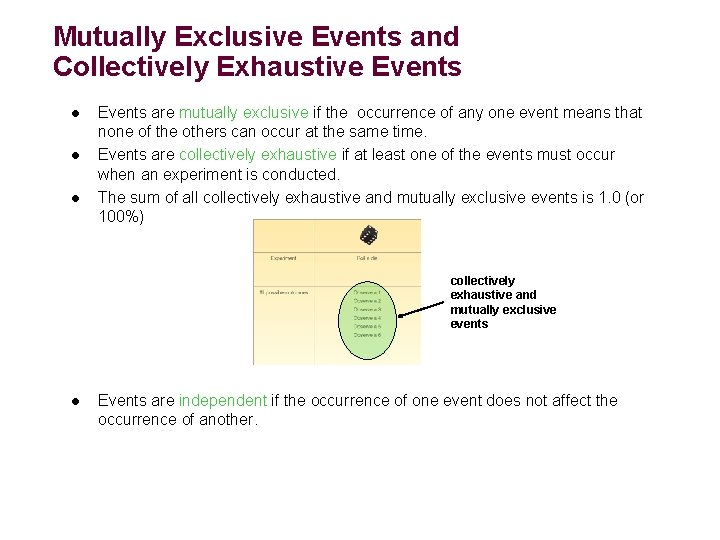Mutually Exclusive Events and Collectively Exhaustive Events l l l Events are mutually exclusive if the occurrence of any one event means that none of the others can occur at the same time. Events are collectively exhaustive if at least one of the events must occur when an experiment is conducted. The sum of all collectively exhaustive and mutually exclusive events is 1. 0 (or 100%) collectively exhaustive and mutually exclusive events l Events are independent if the occurrence of one event does not affect the occurrence of another.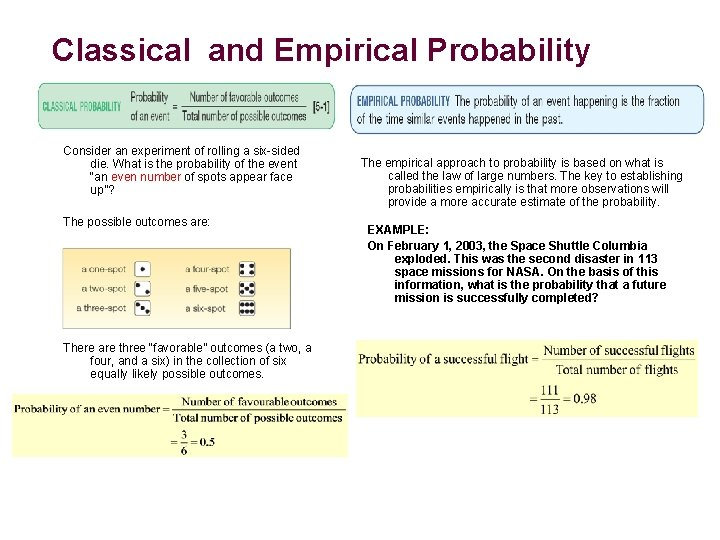Classical and Empirical Probability Consider an experiment of rolling a six-sided die. What is the probability of the event “an even number of spots appear face up”? The possible outcomes are: There are three “favorable” outcomes (a two, a four, and a six) in the collection of six equally likely possible outcomes. The empirical approach to probability is based on what is called the law of large numbers. The key to establishing probabilities empirically is that more observations will provide a more accurate estimate of the probability. EXAMPLE: On February 1, 2003, the Space Shuttle Columbia exploded. This was the second disaster in 113 space missions for NASA. On the basis of this information, what is the probability that a future mission is successfully completed?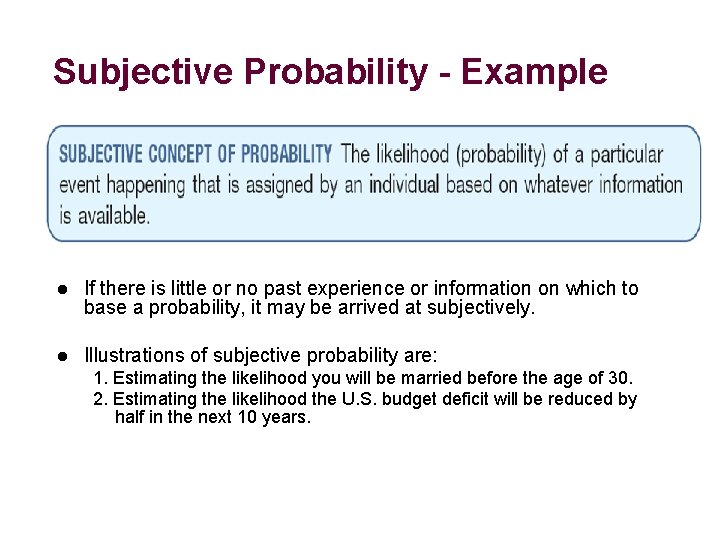Subjective Probability - Example l If there is little or no past experience or information on which to base a probability, it may be arrived at subjectively. l Illustrations of subjective probability are: 1. Estimating the likelihood you will be married before the age of 30. 2. Estimating the likelihood the U. S. budget deficit will be reduced by half in the next 10 years.Summary of Types of ProbabilityRules of Addition EXAMPLE: Rules of Addition l Special Rule of Addition - If two events A and B are mutually exclusive, the probability of one or the other event’s occurring equals the sum of their probabilities. P(A or B) = P(A) + P(B) l An automatic Shaw machine fills plastic bags with a mixture of beans, broccoli, and other vegetables. Most of the bags contain the correct weight, but because of the variation in the size of the beans and other vegetables, a package might be underweight or overweight. A check of 4, 000 packages filled in the past month revealed: The General Rule of Addition - If A and B are two events that are not mutually exclusive, then P(A or B) is given by the following formula: P(A or B) = P(A) + P(B) - P(A and B) What is the probability that a particular package will be either underweight or overweight? P(A or C) = P(A) + P(C) =. 025 +. 075 =. 10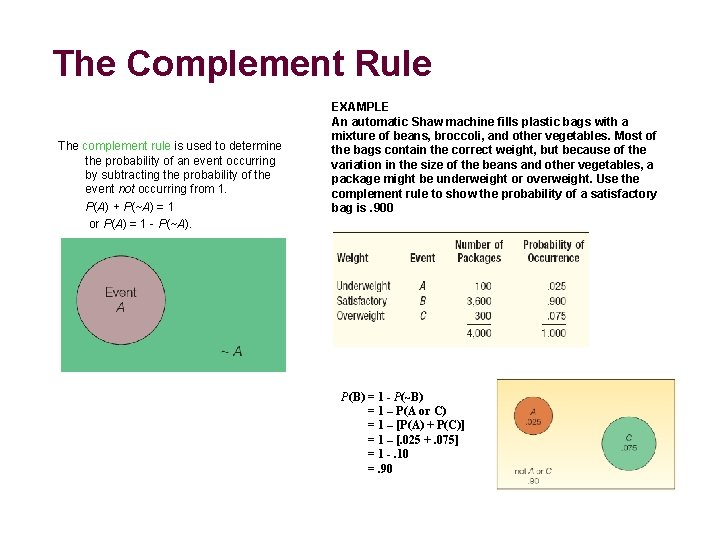The Complement Rule The complement rule is used to determine the probability of an event occurring by subtracting the probability of the event not occurring from 1. P(A) + P(~A) = 1 or P(A) = 1 - P(~A). EXAMPLE An automatic Shaw machine fills plastic bags with a mixture of beans, broccoli, and other vegetables. Most of the bags contain the correct weight, but because of the variation in the size of the beans and other vegetables, a package might be underweight or overweight. Use the complement rule to show the probability of a satisfactory bag is. 900 P(B) = 1 - P(~B) = 1 – P(A or C) = 1 – [P(A) + P(C)] = 1 – [. 025 +. 075] = 1 -. 10 =. 90The General Rule of Addition and Joint Probability The Venn Diagram shows the result of a survey of 200 tourists who visited Florida during the year. The survey revealed that 120 went to Disney World, 100 went to Busch Gardens and 60 visited both. JOINT PROBABILITY A probability that measures the likelihood two or more events will happen concurrently. What is the probability a selected person visited either Disney World or Busch Gardens? P(Disney or Busch) = P(Disney) + P(Busch) - P(both Disney and Busch) = 120/200 + 100/200 – 60/200 =. 60 +. 50 –. 30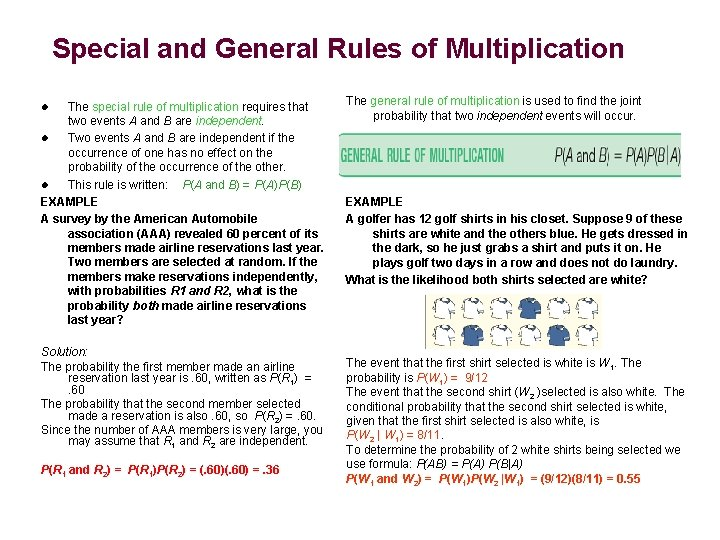Special and General Rules of Multiplication The special rule of multiplication requires that two events A and B are independent. l Two events A and B are independent if the occurrence of one has no effect on the probability of the occurrence of the other. l This rule is written: P(A and B) = P(A)P(B) EXAMPLE A survey by the American Automobile association (AAA) revealed 60 percent of its members made airline reservations last year. Two members are selected at random. If the members make reservations independently, with probabilities R 1 and R 2, what is the probability both made airline reservations last year? l Solution: The probability the first member made an airline reservation last year is. 60, written as P(R 1) =. 60 The probability that the second member selected made a reservation is also. 60, so P(R 2) =. 60. Since the number of AAA members is very large, you may assume that R 1 and R 2 are independent. P(R 1 and R 2) = P(R 1)P(R 2) = (. 60) =. 36 The general rule of multiplication is used to find the joint probability that two independent events will occur. EXAMPLE A golfer has 12 golf shirts in his closet. Suppose 9 of these shirts are white and the others blue. He gets dressed in the dark, so he just grabs a shirt and puts it on. He plays golf two days in a row and does not do laundry. What is the likelihood both shirts selected are white? The event that the first shirt selected is white is W 1. The probability is P(W 1) = 9/12 The event that the second shirt (W 2 )selected is also white. The conditional probability that the second shirt selected is white, given that the first shirt selected is also white, is P(W 2 | W 1) = 8/11. To determine the probability of 2 white shirts being selected we use formula: P(AB) = P(A) P(B|A) P(W 1 and W 2) = P(W 1)P(W 2 |W 1) = (9/12)(8/11) = 0. 55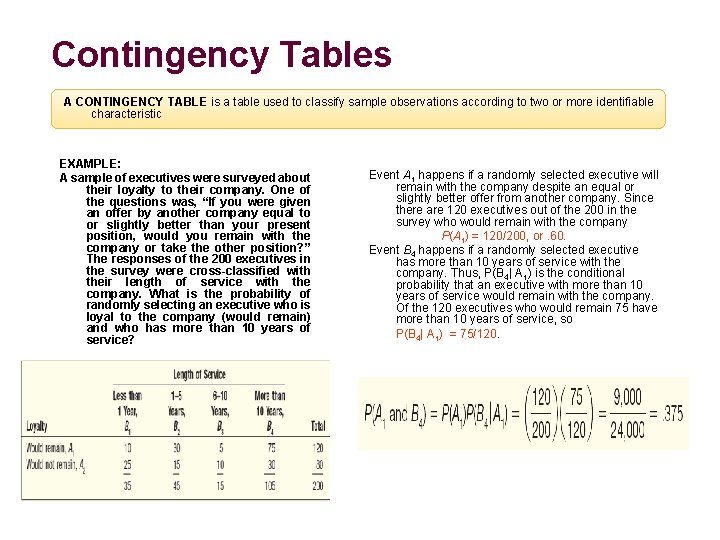Contingency Tables A CONTINGENCY TABLE is a table used to classify sample observations according to two or more identifiable characteristic EXAMPLE: A sample of executives were surveyed about their loyalty to their company. One of the questions was, “If you were given an offer by another company equal to or slightly better than your present position, would you remain with the company or take the other position? ” The responses of the 200 executives in the survey were cross-classified with their length of service with the company. What is the probability of randomly selecting an executive who is loyal to the company (would remain) and who has more than 10 years of service? Event A 1 happens if a randomly selected executive will remain with the company despite an equal or slightly better offer from another company. Since there are 120 executives out of the 200 in the survey who would remain with the company P(A 1) = 120/200, or. 60. Event B 4 happens if a randomly selected executive has more than 10 years of service with the company. Thus, P(B 4| A 1) is the conditional probability that an executive with more than 10 years of service would remain with the company. Of the 120 executives who would remain 75 have more than 10 years of service, so P(B 4| A 1) = 75/120.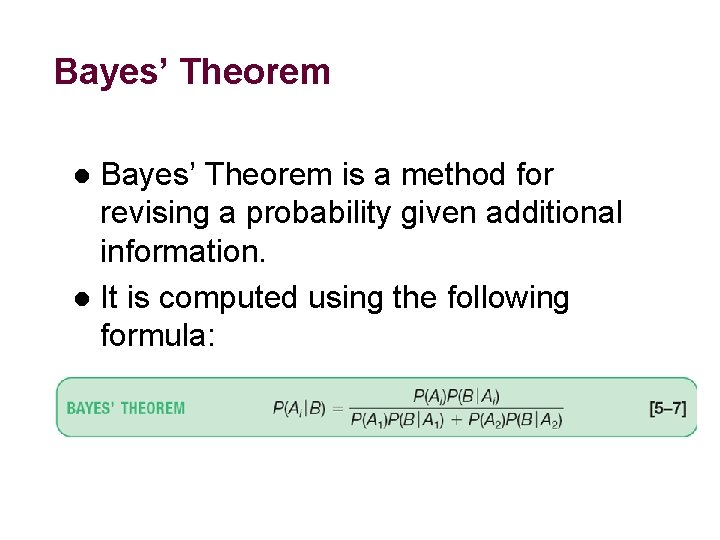Bayes’ Theorem is a method for revising a probability given additional information. l It is computed using the following formula: l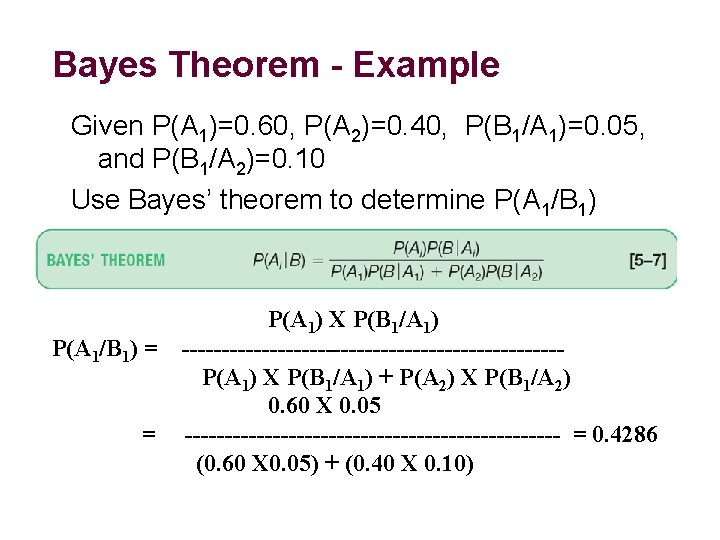Bayes Theorem - Example Given P(A 1)=0. 60, P(A 2)=0. 40, P(B 1/A 1)=0. 05, and P(B 1/A 2)=0. 10 Use Bayes’ theorem to determine P(A 1/B 1) = = 13 P(A 1) X P(B 1/A 1) ------------------------P(A 1) X P(B 1/A 1) + P(A 2) X P(B 1/A 2) 0. 60 X 0. 05 ------------------------ = 0. 4286 (0. 60 X 0. 05) + (0. 40 X 0. 10)